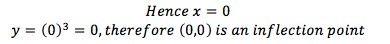top of page#### Inflection Points

###### How to Determine an Inflection Point

An inflection point is a point that when substituted in the second derivative, equals to 0.

Worked Example:

In this example, we will try to find the inflection point of the equation y=x^3. ​

Step 1: Find the Double Derivative (Differentiate Two Times)

###### Horizontal Point of InflectionA horizontal point of inflection is a point with a gradient of 0 and has a positive or negative gradient on both sides. A horizontal point of inflection is also an inflection point as the double derivate will equal to 0 at its co-ordinates.

A horizontal point of inflection with both sides being positive is a positive horizontal point of inflection. A horizontal point of inflection with both sides being negative is a negative horizontal point of inflection.

We can use the sign test to prove that (0,0) is a horizontal point of inflection in the curve y=x^3.### Applications of Differentiation

Step 2: Make the Double Derive Equal 0 and Solve for X

Step 2: After finding x, use the equation to find y-co-ordinates of the inflection point.You can also use the sign test to determine a horizontal point of inflection.###### Regular Inflection Points

Inflection points that are not horizontal points of inflection represent the largest gradient between two stationary points. It represents the point where the concavity changes, such as a transition from a minimum turning point to a maximum turning point.

At the inflection point, the gradient stops decreasing or increasing in value between two inflection points.

Below shows the graph of y=3x^3-2x and its inflection point of (0,0).Worked Example of Determining (0,0) as the Inflection Pointbottom of page